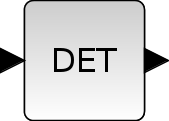Scilab Home page | Wiki | Bug tracker | Forge | Mailing list archives | ATOMS | File exchange
Change language to: English - Français - Português - 日本語 -

See the recommended documentation of this function

# MATDET

Matrix Determinant

### Block Screenshot### Description

The MATDET outputs the determinant of a square input matrix. If the input is:

A=[A11 A12 A13;A21 A22 A23;A31 A32 A33]

then the output of the block has the form of:

y=A11*(A22*A33-A23*A32)-A12*(A21*A33-A23*A31)+A13*(A21*A32-A22*A31).

The equivalent function of MATDET in Scilab is det.

### Dialog box• Datatype(1=real double 2=Complex)

It indicates the type of the output. It support only the two types double (1) and complex (2). If we input another entry in this label xcos will print the message "Datatype is not supported".

Properties : Type 'vec' of size 1.

### Example

```U=[1 0 63;2 -2 5;9 9 4]
y=2215```

### Default properties

• always active: no

• direct-feedthrough: yes

• zero-crossing: no

• mode: no

• regular inputs:

- port 1 : size [-1,-1] / type 1

• regular outputs:

- port 1 : size [1,1] / type 1

• number/sizes of activation inputs: 0

• number/sizes of activation outputs: 0

• continuous-time state: no

• discrete-time state: no

• object discrete-time state: no

• name of computational function: mat_det

### Interfacing function

• SCI/modules/scicos_blocks/macros/MatrixOp/MATDET.sci

### Computational function

• SCI/modules/scicos_blocks/src/c/mat_det.c

• SCI/modules/scicos_blocks/src/c/matz_det.c

### Determinant example: det([tan(x) 0 ; 0 cos(x)]) = sin(x)